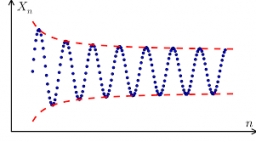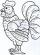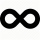# Quotient and division

What is the quotient of 2/9 and 1/8?

k =  1.7778

### Step-by-step explanation:Did you find an error or inaccuracy? Feel free to write us. Thank you!Tips to related online calculators
Need help to calculate sum, simplify or multiply fractions? Try our fraction calculator.

## Related math problems and questions:

• Quotient 3If the quotient of 8/13 and 2 is subtracted from the product of 1 3/4 and 8/21, what is the difference?
• The quotientThe quotient of g and 55 is the same as 279. What is g?
• Quotient and productIf the quotient of [8/5 divided by 8/10] is added to the product of [8/14 x 7/12 x 3/8], what is the sum?
• Quotient and divisionFind the quotient of 3/4 and 1/4.
• A quotientWhat is the quotient of 3/10 divided by 2/4 as a fraction?
• QuotientDetermine the quotient (q) and the remainder (r) from division numbers 100 and 8. Take the test of correctness.
• What is 11What is the quotient of Three-fifths and 1 Over 10?
• Find the 11Find the quotient of 229.12 and 12.32
• SixteenSixteen minus the quotient of 12 and what number is thirteen?
• Numbers divisionWith what number should be divided mixed number 2 3/4 to get 11/12?
• QuotientFind quotient before the bracket - the largest divisor 51 a + 34 b + 68 121y-99z-33
• How many 16How many three-tenths are there in two and one-fourths?
• Evaluate 12Evaluate and simplify 9 divided by 7/6.
• Division by zeroFraction 5 by 2. if 3 is added to numerator and 2 is subtracted from the denominator then the new fraction is:
• Divide 11Divide the product of 4 and 5/8 by 1 1/2. Write your answer as a mixed number.
• The quotient 2The quotient of the quantity k minus 10 and m
• Two numbers and its productThe product of two numbers are 2/3. If on of them is 1/10, what is the other?